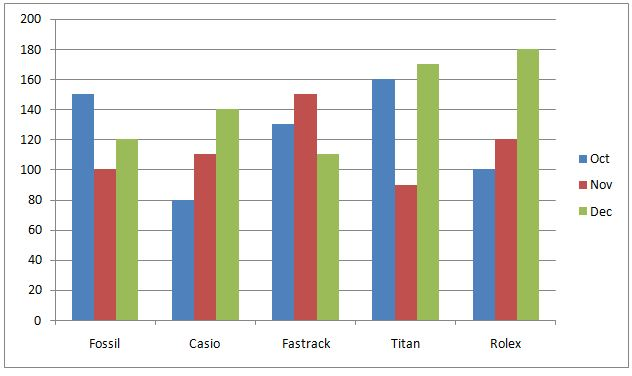# CWC/FCI Prelims 2019 – Quantitative Aptitude Questions (Day-11)

Dear Readers, Exam Race for the Year 2019 has already started, To enrich your preparation here we are providing new series of Practice Questions on Quantitative Aptitude – Section for CWC/FCI Exam. Aspirants, practice these questions on a regular basis to improve your score in aptitude section. Start your effective preparation from the right beginning to get success in upcoming CWC/FCI Exam.

[WpProQuiz 5496]

Directions (Q. 1 – 5): In the following questions, two equations I and II are given. You have to solve both the equations and give answer as,

a) If x > y

b) If x ≥ y

c) If x < y

d) If x ≤ y

e) If x = y or the relation cannot be established

1)

I) 3x2 – 10x – 77 = 0

II) 4y2 + 2y – 56 = 0

2)

I) 3x – 4y = 4

II) 2x + 5y = 18

3)

I) x2 – 52 = 168 ÷ 3

II) y2 – 7x + 12 = 0

4)

I) 2x2 + 10x + 12 = 0

II) 3y2 + 11y + 10 = 0

5)

I) x2 – 6x – 27 = 0

II) y2 – 7y – 33 = 0

Directions (Q. 6 – 10): Study the following information carefully and answer the given questions:

The following bar graph shows the total number of different types of brand watches sold in 3 different months in a certain city.6) Find the ratio between the total number of Fossil watches to that of Casio watches sold in all the given months together?

a) 12: 7

b) 37: 33

c) 53: 49

d) 115: 107

e) None of these

7) Find the difference between the average number of watches sold in the month of Oct to that of Dec?

a) 20

b) 30

c) 50

d) 40

e) None of these

8) Find the average number of watches sold in the month of Nov?

a) 136

b) 128

c) 102

d) 114

e) None of these

9) Total number of Fastrack watches sold in all the given months together is what percentage of total number of Rolex watches sold in all the given months together?

a) 88.25 %

b) 97.5 %

c) 92.25 %

d) 76.75 %

e) None of these

10) Total number of watches sold in the month of Dec is approximately what percentage less than total number of Fossil and Rolex watches sold in all the given months together?

a) 15 % more

b) 15 % less

c) 6 % less

d) 6 % more

e) 22 % less

I) 3x2 – 10x – 77 = 0

3x2 – 21x + 11x – 77 = 0

3x (x – 7) + 11 (x – 7) = 0

(3x + 11) (x – 7) = 0

X = -11/3, 7 = -3.667, 7

II) 4y2 + 2y – 56 = 0

4y2 + 16y – 14y – 56 = 0

4y (y + 4) – 14 (y + 4) = 0

(4y – 14) (y + 4) = 0

Y = 14/4, -4 = 3.5, -4

Can’t be determined

3x – 4y = 4 —> (1)

2x + 5y = 18 —> (2)

By solving the equation (1) and (2), we get,

X = 4, y = 2

X > y

I) x2 – 52 = 168 ÷ 3

(x2 – 25) = (168/3)

x2 = 56 + 25 = 81

x = 9, -9

II) y2 – 7x + 12 = 0

(y – 4) (y – 3) = 0

Y = 4, 3

Can’t be determined

I) 2x2 + 10x + 12 = 0

2x2 + 4x + 6x + 12 = 0

2x (x + 2) + 6(x + 2) = 0

(2x + 6) (x + 2) = 0

X = -6/2, -2 = -3, -2

II) 3y2 + 11y + 10 = 0

3y2 + 6y + 5y + 10 = 0

3y (y + 2) + 5 (y + 2) = 0

(3y + 5) (y + 2) = 0

Y = -5/3, -2 = -1.667, -2

X ≤ y

I) x2 – 6x – 27 = 0

(x – 9) (x + 3) = 0

X = 9, -3

II) y2 – 7y – 33 = 0

(y – 11) (y + 3) = 0

Y = 11, -3

Can’t be determined

Directions (6-10):

The total number of Fossil watches sold in all the given months together

= > 150 + 100 + 120 = 370

The total number of Casio watches sold in all the given months together

= > 80 + 110 + 140 = 330

Required ratio = 370: 330 = 37: 33

The average number of watches sold in the month of Oct

= > (150 + 80 + 130 + 160 + 100)/5 = 620/5 = 124

The average number of watches sold in the month of Dec

= > (120 + 140 + 110 + 170 + 180)/5 = 720/5 = 144

Required difference = 144 – 124 = 20

The average number of watches sold in the month of Nov

= > (100 + 110 + 150 + 90 + 120)/5

= > 570/5 = 114

Total number of Fastrack watches sold in all the given months together

= > 130 + 150 + 110 = 390

Total number of Rolex watches sold in all the given months together

= > 100 + 120 + 180 = 400

Required % = (390/400)*100 = 97.5 %

Total number of watches sold in the month of Dec

= > 120 + 140 + 110 + 170 + 180 = 720

Total number of Fossil and Rolex watches sold in all the given months together

= > 150 + 100 + 120 + 100 + 120 + 180 = 770

Required % = [(770 – 720)/770]*100 = 6.49 % = 6 % less